Next: IR Scattering from Mie Up: Infrared Spectrum Previous: IR Radiative Transfer

### FASCOD3P Model

An atmospheric radiative transfer model is required to determine the downwelling clear sky radiance,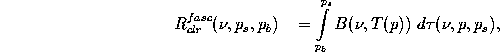upwelling clear sky radiance, including terrestrial contribution,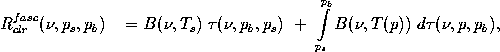and transmission,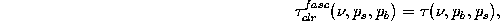below the cloud base. The Air Force Philips laboratory line-by-line transmission model, Fast Atmospheric Signature Code (FASCOD3P) (Clough et al., 1986), is used for this purpose. Knowledge of the clear sky radiance and transmission effectively projects the ground-based downwelling measured radiance to cloud base.

The model utilizes the Air Force Geophysics Laboratory HITRAN database (Rothman et al., 1987) of atmospheric transmission lines and a local radiosonde profile to calculate radiance and transmission of the atmosphere. The radiosonde is used to obtain temperature and moisture profiles. A number of additional parameters (e.g., spectral information, additional atmospheric data, path characteristics, and output format) are also required in the FASCOD3P input file. Appendix C shows a typical FASCOD3P input file and provides an explanation of the individual parameters.

Figure 2 illustrates data for a radiosonde launched on 17 November 1994, 00:00 UTC (16 November, 18:00 CST) in Madison, WI during an evening of scattered cirrus. Temperature and dewpoint temperature are shown as a function of altitude. The individual data points indicate levels that were used in the FASCOD3P calculations. These points were chosen to preserve the measured vertical structure with a reduced number of levels, to satisfy model constraints. Also given are the lidar measured cloud boundaries, which agree with the low dewpoint depression in the radiosonde measurements.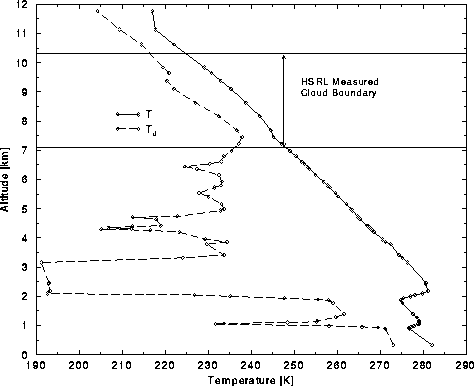Figure 2: Radiosonde data for Madison, WI on 17 November 1994, 00:00 UTC. Points indicate FASCOD3P levels, where the solid line indicates temperature and the dashed line dewpoint temperature.

The application of FASCOD3P in the present context results in two primary limitations. The first is the proper representation of the water vapor continuum (Grant, 1990; Cachorro et al., 1987; Clough, 1995; Theriault et al., 1994). The second is the ability to account for the presence of lower level aerosols (Volz, 1973). As a result, the FASCOD3P radiance prediction may differ by as much as 40 percent from the AERI measured values. Figure 3 provides an example which shows radiance differences which correspond to brightness temperature differences of up to 15 K. A correction, referred to as the Gamma technique (Smith et al., 1993), can be applied to the FASCOD3P calculated transmittance until the radiance values agree with clear sky AERI measurements. A simultaneous lidar observation ensures the AERI observation is indeed clear.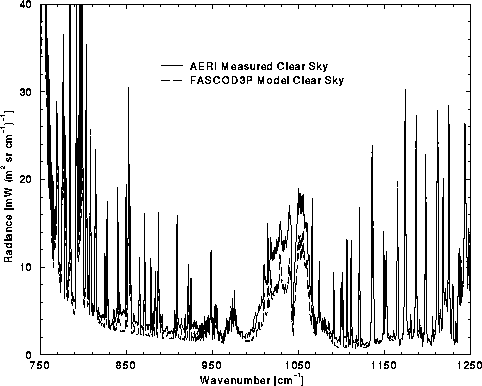Figure 3: AERI measured and FASCOD3P calculated clear sky atmospheric column radiance for data acquired at Madison, WI on 17 November 1994, 00:00 UTC. The upper solid-line represents AERI data while the lower dashed-line is the model calculated radiance.

Cases which lack a clear sky observation present an additional shortcoming to this technique. Gamma values from another case must be used when this occurs, similar to the case in question. One approach would be to obtain a database of measurements based on atmospheric conditions and season, which could be applied to this situation. Fortunately, this predicament is not a factor in the data to be presented in this thesis. Furthermore, the AERI is typically operated in an environment which allows continuous data acquisition, given acceptable weather conditions. This would increase the likelihood of observing a clear sky data point near the time of interest.

AERI measured column radiance,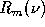, differs from the FASCOD3P calculation by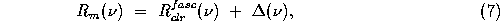where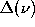is the FASCOD3P derived radiance error. Although a correction is applied to adjust the model radiance to match the measured radiance, knowledge ofis required to correct for the model determined radiance from the surface to cloud base. Equation 7 relates to the total column radiance, however it is assumed that thecontribution occurs in the lower atmosphere. Therefore, the same bias is applied to the model derived radiance from surface to cloud base,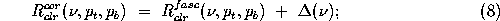where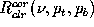represents the corrected model radiance.

The Gamma correction is achieved by linearly scaling the FASCOD3P derived optical depth as a function of wavenumber,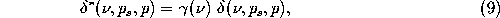where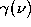is the scaling factor,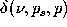is the uncorrected spectral optical depth, and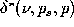is the corrected spectral optical depth.

A forward iteration inis executed until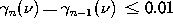, using the following method (Smith et al., 1993):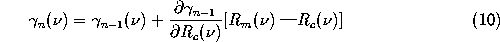where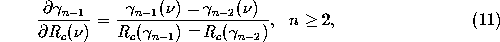and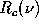is the corrected radiance based on the current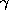corrected transmittance. The iteration is seeded with an initialvalue of either 0.95 or 1.05, dependent upon the sign of the original difference between the measured and calculated radiance. Figure 4 illustrates the resultantspectrum for the radiance difference specified in Figure 3.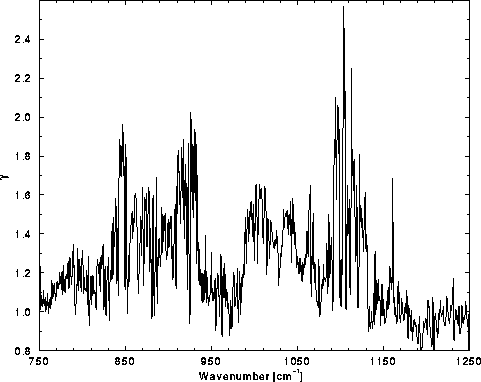Figure 4: Gamma spectrum for AERI measured clear sky and FASCOD3P calculated radiance difference shown in Figure 3. The spectral Gamma values scale the FASCOD3P infrared optical depth to account for the model radiance difference.

The Gamma technique provides a means for correcting the atmospheric transmission through radiance observations. However, the approach adjusts the column transmissivity based on spectral selectivity and does not account for vertical differences in atmospheric constituents. This becomes a problem when vertical resolution is significant to the calculations; but should not be a factor in these calculations, which require a single transmissivity.

The downwelling radiance from the region above the cloud is assumed to be small in all calculations. FASCOD3P can be utilized to show that the radiance contribution is negligible for the atmosphere above cirrus level clouds. This is accomplished by comparing the FASCOD3P calculated downwelling radiance from the earth surface to an altitude of 9 km against a similar calculation to 15 km. It should be noted that this comparison will represent a maximum difference, because the model does not include the effects of cirrus which would further attenuate the upper atmospheric source while increasing the total radiance measured by the instrument.

Figure 5 shows the fractional difference in FASCOD3P calculated downwelling radiance using an upper limit of 15 km versus 9 km, which yields the percent radiance reaching the surface from an atmospheric layer between 9 and 15 km. The upper limit of 15 km was chosen based on cirrus cloud top altitude. A radiance contribution due to ozone, between 950 and 1060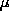m, occurs beyond this altitude, but it not a factor when using data within the chosen microwindows. Calculations are based on local radiosonde data at Madison, WI on 17 November 1994, 00:00 UTC, shown in Figure 2. HO and Oare the primary features, while the remainder of the spectral region contributes a negligible radiance.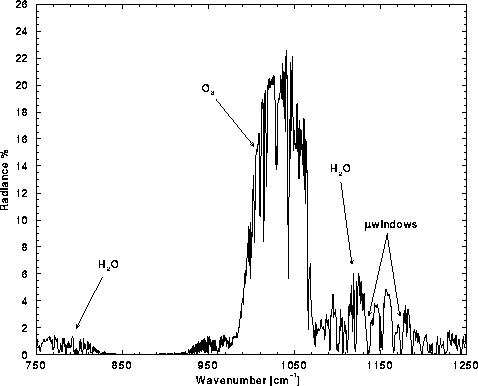Figure 5: Downwelling fractional radiance difference for altitudes 9 through 15 km signifies a negligible atmospheric radiance contribution above cloud top for spectral regions outside absorption lines; based on FASCOD3P model simulations.Next: IR Scattering from Mie Up: Infrared Spectrum Previous: IR Radiative Transfer

Daniel DeSlover
Sun Aug 11 10:02:40 CDT 1996